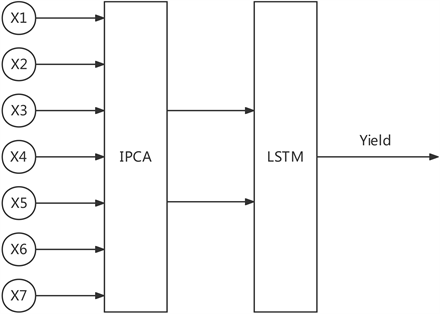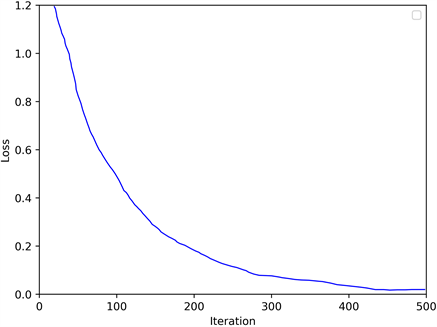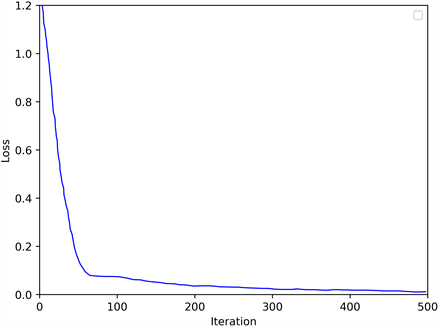#### 期刊菜单

Research on LSTM Rice Yield Prediction Model Based on Improved Principal Component Analysis
DOI: 10.12677/SEA.2022.113048, PDF, HTML, XML, 下载: 86  浏览: 197

Abstract: As a major agricultural country, agriculture has always been an important cornerstone of our country’s economic development. As the main food crop in our country, with the continuous growth of the population, the demand for rice is also rising. Therefore, the prediction of rice production is very important for the development and construction of agriculture and guarantee of food security. Long short-term memory (LSTM) recurrent neural network has a good application prospect in the field of crop yield prediction because it can not only deal with the nonlinear relationship between various factors, but also is suitable for dealing with the prediction problem of time series data. In this paper, a LSTM recurrent neural network based on improved principal component analysis is proposed, which reduces the data dimension of the input of the neural network, aiming to improve the convergence speed of neural network and eliminate the information redundancy caused by the correlation between the input data, thereby improving prediction accuracy.

1. 引言

2. 相关工作

2.1. LSTM循环神经网络

LSTM是一种特殊的循环神经网络(RNN)，通过在标准RNN中引入门控单元的概念来解决标准RNN中可能存在的梯度消失问题 。LSTM循环神经网络的基本结构如图1所示。其中 ${f}_{t}$ 为遗忘门， ${i}_{t}$ 为输入门， ${o}_{t}$ 为输出门， ${\stackrel{˜}{c}}_{t}$ 是标准RNN单元中的结构。 ${x}_{t}$ 表示一个输入节点，用来对应一个特征参数， ${h}_{t}$ 表示t时刻该单元的信息输出， ${C}_{t}$ 表示t时刻记忆单元的状态。 $\sigma$ 一般选择Sigmoid作为激励函数，主要起门控作用，由于Sigmoid函数的输出为0-1，当输出接近0或1时符合物理意义上的关和开。tanh函数作为生成候选记忆单元c的选项，因其输出在−1到1之间，符合多数场景下中心为0的特征分布，且梯度(求导)在接近0处的收敛速度快于Sigmoid函数。Figure 1. Basic structure of LSTM recurrent neural network

${f}_{t}=\sigma \left({W}_{xf}{x}_{t}+{W}_{hf}{h}_{t-1}+{b}_{f}\right)$ (1)

${i}_{t}=\sigma \left({W}_{xi}{x}_{t}+{W}_{hi}{h}_{t-1}+{b}_{i}\right)$ (2)

${o}_{t}=\sigma \left({W}_{xo}{x}_{t}+{W}_{ho}{h}_{t-1}+{b}_{o}\right)$ (3)

${\stackrel{˜}{c}}_{t}=\mathrm{tanh}\left({W}_{x\stackrel{˜}{c}}{x}_{t}+{W}_{h\stackrel{˜}{c}}{h}_{t-1}+{b}_{\stackrel{˜}{c}}\right)$ (4)

${C}_{t}={C}_{t-1}\ast {f}_{i}+{\stackrel{˜}{c}}_{t}\ast {i}_{t}$ (5)

${h}_{t}={o}_{t}\ast \mathrm{tanh}\left({C}_{t}\right)$ (6)

2.2. 主成分分析

$\begin{array}{l}{Z}_{1}={{a}^{\prime }}_{1}y={a}_{11}{Y}_{1}+{a}_{12}{Y}_{2}+{a}_{13}{Y}_{3}+\cdots +{a}_{1p}{Y}_{p}\\ {Z}_{2}={{a}^{\prime }}_{2}y={a}_{21}{Y}_{1}+{a}_{22}{Y}_{2}+{a}_{13}{Y}_{3}+\cdots +{a}_{2p}{Y}_{p}\\ \text{\hspace{0.17em}}\text{\hspace{0.17em}}\text{\hspace{0.17em}}\text{\hspace{0.17em}}\text{\hspace{0.17em}}\text{\hspace{0.17em}}\text{\hspace{0.17em}}\text{\hspace{0.17em}}\text{\hspace{0.17em}}\text{\hspace{0.17em}}\text{\hspace{0.17em}}\text{\hspace{0.17em}}\text{\hspace{0.17em}}\text{\hspace{0.17em}}\text{\hspace{0.17em}}\text{\hspace{0.17em}}\text{\hspace{0.17em}}\text{\hspace{0.17em}}\text{\hspace{0.17em}}\text{\hspace{0.17em}}\text{\hspace{0.17em}}\text{\hspace{0.17em}}\text{\hspace{0.17em}}\text{\hspace{0.17em}}\text{\hspace{0.17em}}\text{\hspace{0.17em}}\text{\hspace{0.17em}}\text{\hspace{0.17em}}\text{\hspace{0.17em}}\text{\hspace{0.17em}}\text{\hspace{0.17em}}\text{\hspace{0.17em}}⋮\\ {Z}_{p}={{a}^{\prime }}_{p}y={a}_{p1}{Y}_{1}+{a}_{p2}{Y}_{2}+{a}_{p3}{Y}_{3}+\cdots +{a}_{pp}{Y}_{p}\end{array}$

${Z}_{j}={{e}^{\prime }}_{j}y={e}_{j1}{Y}_{1}+{e}_{j2}{Y}_{2}+{e}_{j3}{Y}_{3}+\cdots +{e}_{jp}{Y}_{p},j=1,2,\cdots ,p$ (7)

$\mathrm{var}\left({Z}_{j}\right)={{e}^{\prime }}_{j}\Sigma {e}_{j}={\lambda }_{j}$ (8)

$\mathrm{cov}\left({Z}_{j},{Z}_{k}\right)={{e}^{\prime }}_{j}\Sigma {e}_{k}=0$ (9)

${\sum }_{j=1}^{p}\mathrm{var}\left({Z}_{j}\right)={\sum }_{j=1}^{p}\mathrm{var}\left({Y}_{j}\right)$ (10)

${W}_{j}=\left({Y}_{j}-{u}_{i}\right)/\sqrt{{\sigma }_{jj}}$ (11)

2.3. 主成分分析存在的问题及改进

$\text{CV}=\frac{\sigma }{\mu }$ (12)

${y}_{ij}=\frac{{x}_{ij}}{\stackrel{¯}{{x}_{j}}}$ (13)

${x}_{j}=\frac{1}{n}{\sum }_{k=1}^{n}{x}_{kj}$ (14)

${c}_{ij}=\frac{1}{n}{\sum }_{k=1}^{n}\left({y}_{ki}-\stackrel{¯}{{y}_{i}}\right)\left({y}_{kj}-\stackrel{¯}{{y}_{j}}\right)$ (15)

${c}_{ij}=\frac{1}{n}{\sum }_{k=1}^{n}\left(\frac{{x}_{ki}}{\stackrel{¯}{{x}_{i}}}-1\right)\left(\frac{{x}_{kj}}{\stackrel{¯}{{x}_{j}}}-1\right)$ (16)

${c}_{ij}=\frac{\frac{1}{n}{\sum }_{k=1}^{n}\left({x}_{ki}-\stackrel{¯}{{x}_{i}}\right)\left({x}_{kj}-\stackrel{¯}{{x}_{j}}\right)}{\stackrel{¯}{{x}_{i}}\cdot \stackrel{¯}{{x}_{j}}}$ (17)

${r}_{ij}=\frac{{c}_{ij}}{\sqrt{{c}_{ii}}\cdot \sqrt{{c}_{jj}}}$ (18)

${r}_{ij}=\frac{{s}_{ij}}{\sqrt{{s}_{ii}}\cdot \sqrt{{s}_{jj}}}$ (19)

$\frac{{s}_{ij}}{\sqrt{{s}_{ii}}\cdot \sqrt{{s}_{jj}}}={\rho }_{ij}$，因此该标准化也不会丢失原始变量的相关系数信息，综上所述，该标准化方法可以更完整地刻画原始变量的信息。

3. 模型构建Figure 2. Structure of LSTM based on PCA

3.1. 主成分分析相关计算

3.2. 神经网络模型构建

4. 实验结果及分析

4.1. 数据集

4.2. 相关参数计算及数据预处理Table 2. KMO and Bartlett’s test of sphericity

$\text{F}1=-0.781{X}_{1}+0.796{X}_{2}+0.949{X}_{3}+0.991{X}_{4}+0.925{X}_{5}+0.058{X}_{6}+0.076{X}_{7}$ (20)

$\text{F}2=0.490{X}_{1}+0.325{X}_{2}+{0.1573}_{1}+0.023{X}_{4}-0.192{X}_{5}+0.973{X}_{6}+0.963{X}_{7}$ (21)

4.3. 模型对比

$\text{RMSE}\left(X,h\right)=\sqrt{\frac{1}{n}{\sum }_{i=1}^{n}{\left(h\left({x}_{i}\right)-{y}_{i}\right)}^{2}}$ (22)

$\text{MAPE}\left(X,h\right)=100%×\frac{1}{n}{\sum }_{i=1}^{n}|h\left({x}_{i}\right)-{y}_{i}|$ (23)Figure 3. Loss of BP neural network on training setFigure 4. Loss of IPCA-LSTM on training setTable 5. System results of different models on the dataset

5. 结论

  Guo, Y., Fu, Y., Hao, F., Zhang, X., Wu, W., Jin, X., et al. (2021) Integrated Phenology and Climate in Rice Yields Pre-diction Using Machine Learning Methods. Ecological Indicators, 120, Article ID: 106935. https://doi.org/10.1016/j.ecolind.2020.106935  王雨晨. 基于灰色模型的江西水稻产量预测研究[J]. 粮食科技与经济, 2020, 45(4): 29-30. https://doi.org/10.16465/j.gste.cn431252ts.20200404  李红艳, 徐建强, 许甫金, 肖玉苹, 沈足金, 张乐平, 方明. 气象因素对水稻产量的影响及预测模型的建立[J]. 浙江农业科学, 2018, 59(7): 1104-1107+1110. https://doi.org/10.16178/j.issn.0528-9017.20180707  沈陈华. 气象因子对江苏省水稻单产的影响[J]. 生态学报, 2015, 35(12): 4155-4167. https://doi.org/10.5846/stxb201309212315  赵桂涛, 刘中聚, 冯尚宗, 赵理, 王世伟, 娄华敏. 基于气象因素的临沂水稻产量评估预测模型[J]. 江西农业学报, 2016, 28(10): 71-74. https://doi.org/10.19386/j.cnki.jxnyxb.2016.10.16  向昌盛, 张林峰. 灰色理论和马尔可夫相融合的粮食产量预测模型[J]. 计算机科学, 2013, 40(2): 245-248.  薛利红, 曹卫星, 罗卫红. 基于冠层反射光谱的水稻产量预测模型[J]. 遥感学报, 2005, 9(1): 100-105.  Schlenker, W. and Roberts, M.J. (2009) Nonlinear Temperature Effects Indicate Severe Damages to US Crop Yields under Climate Change. Proceedings of the National Academy of Sciences of the United States of America, 106, 15594-15598. https://doi.org/10.1073/pnas.0906865106  汪小旵, 丁为民, 罗卫红, 戴剑锋. 利用BP神经网络对江淮地区梅雨季节现代化温室小气候的模拟与分析[J]. 农业工程学报, 2004, 20(2): 235-238.  任守纲, 刘鑫, 顾兴健, 王浩云, 袁培森, 徐焕良. 基于R-BP神经网络的温室小气候多步滚动预测模型[J]. 中国农业气象, 2018, 39(5): 314-324.  宗宸生, 郑焕霞, 王林山. 改进粒子群优化BP神经网络粮食产量预测模型[J]. 计算机系统应用, 2018, 27(12): 204-209. https://doi.org/10.15888/j.cnki.csa.006651  李灿东. 黑龙江省北部大豆主栽品种产量性状主成分分析[J]. 现代化农业, 2020(12): 4-8.  Borrego, C., Tchepel, O., Costa, A.M., Amorim, J.H. and Miranda, A.I. (2003) Emission and Dispersion Modelling of Lisbon Air Quality at Local Scale. Atmospheric Environment, 37, 5197-5205. https://doi.org/10.1016/j.atmosenv.2003.09.004  Sousa, S.I.V., Martins, F.G., Alvim-Ferraz, M.C.M. and Pe-reira, M.C. (2007) Multiple Linear Regression and Artificial Neural Networks Based on Principal Components to Predict Ozone Concentrations. Environmental Modelling & Software, 22, 97-103. https://doi.org/10.1016/j.envsoft.2005.12.002  肖枝洪, 冉小华. 运用主成分分析法的过程控制和诊断[J]. 重庆理工大学学报(自然科学), 2014, 28(1): 96-101.  梁佐堂, 刘欣涛. 基于主成分分析的手写体数字识别方法研究[J]. 信息技术, 2016(8): 121-124. https://doi.org/10.13274/j.cnki.hdzj.2016.08.030  张驰, 郭媛, 黎明. 人工神经网络模型发展及应用综述[J]. 计算机工程与应用, 2021, 57(11): 57-69.  郭凯维, 郭传超, 史耀凡, 于水. 基于主成分分析的GA-BP神经网络地表下沉系数预测[J]. 北京测绘, 2021, 35(11): 1374-1379. https://doi.org/10.19580/j.cnki.1007-3000.2021.11.003  和树栋. 基于BP神经网络的保护层卸压高度预测方法研究[J]. 煤炭技术, 2020, 39(6): 73-75. https://doi.org/10.13301/j.cnki.ct.2020.06.022  崔巍, 顾冉浩, 陈奔月, 王文. BP与LSTM神经网络在福建小流域水文预报中的应用对比[J]. 人民珠江, 2020, 41(2): 74-84.